# HP - harmonic progression

Determine the 8th term of the harmonic progression 2, 4/3, 1,…

h8 =  0.4444

### Step-by-step explanation:Did you find an error or inaccuracy? Feel free to write us. Thank you!## Related math problems and questions:

• HP - harmonic progressionDetermine the 10th term of the harmonic progression 6,4,3,…
• HP - harmonic progression 2Compute the 16th term of the HP if the 6th and 11th term of the harmonic progression are 10 and 18 respectively.
• Sequence 11What is the nth term of this sequence 1, 1/2, 1/3, 1/4, 1/5?
• Harmonic mean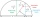If x, y, z form a harmonic progression, then y is the harmonic mean of x and z. Find the harmonic mean of the numbers 6 and 5.
• Insert 7Insert five harmonic means between 3 and 18
• Arithmetic progression 2The 3rd term of an Arithmetic progression is ten more than the first term, while the fifth term is 15 more than the second term. Find the sum of the 8th and 15th terms of the Arithmetic progression if the 7th term is seven times the first term.
• Insert 5Insert five harmonic means between 1/2 and 1/26
• Insert 6Insert four harmonic means between 3/7 and 3/19
• If the 3If the 6th term of a GP is 4 and the 10th is 4/81, find common ratio r.
• Find the 2Find the term independent of x in the expansion of (4x3+1/2x)8
• Harmonic seriesInsert four members between 5/3 and 5/11 to form harmonic series (means).
• What is 10What is the 5th term, if the 8th term is 80 and common ratio r =1/2?
• 76% is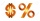76% is between which of the following two numbers. 1) 1/5 & 1/4. 2) 1/3 & 1/2 3) 2/3 & 7/10 4) 3/4 & 5/6
• Simplify 3Simplify mixed numerals expression: 8 1/4- 3 2/5 - (2 1/3 - 1/4) Show your solution.
• Common difference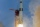The 4th term of an arithmetic progression is 6. If the sum of the 8th and 9th terms is -72, find the common difference.
• Median or middle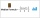The number of hours of television watched per day by a sample of 28 people is given below: 4, 1, 5, 5, 2, 5, 4, 4, 2, 3, 6, 8, 3, 5, 2, 0, 3, 5, 9, 4, 5, 2, 1, 3, 4, 7, 2, 9 What is the median value?
• Insert 3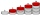Insert five arithmetic progression members between -7 and 3/2.